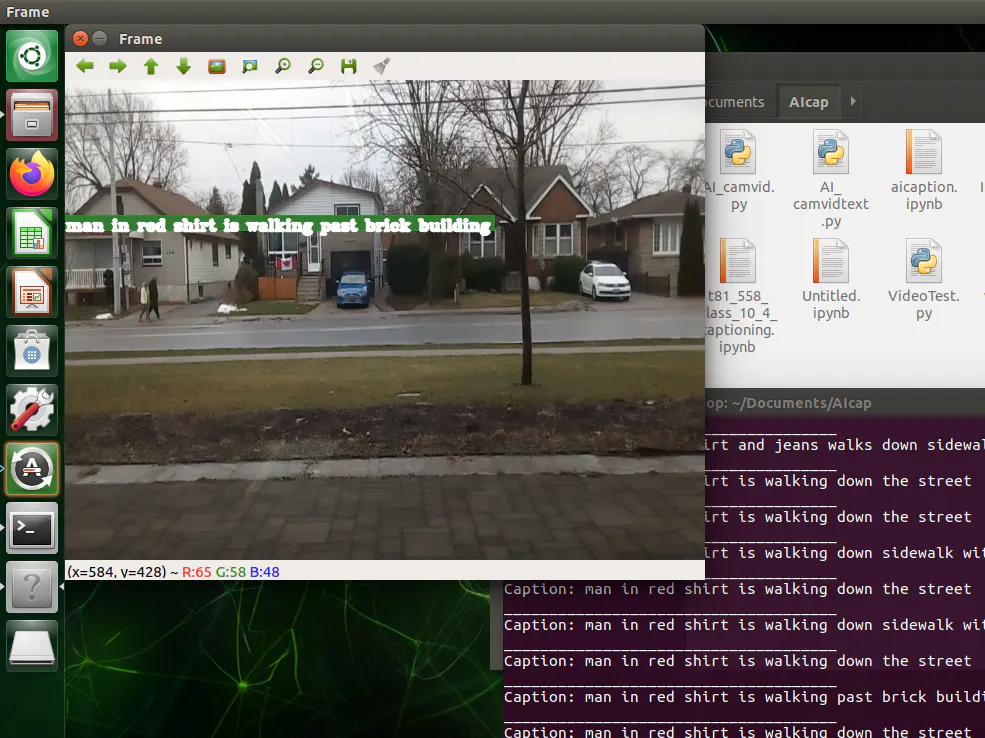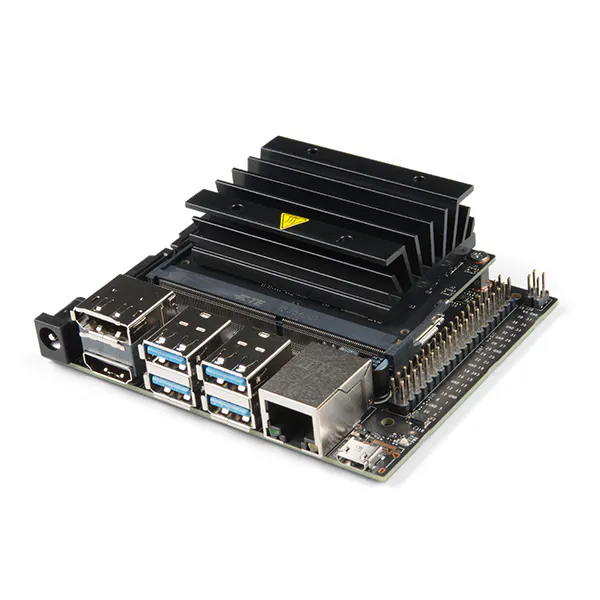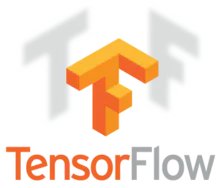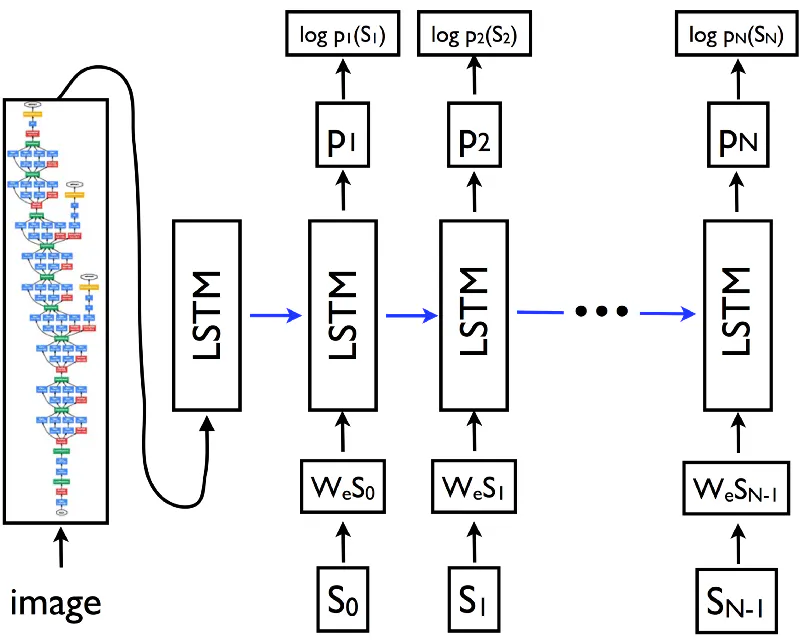# Descriptive AI Camera

Build a camera that automatically describes what it sees using AI captioning.## Things used in this project

### Hardware componentsNVIDIA Jetson Nano Developer Kit
×1
 USB camera
×1

### Software apps and online servicesTensorFlowOpenCV

## Schematics

### Video Captioning netowkr AI network

Video Captioning netowkr top-level architecture## Code

### JetsonNano_ImageCaptioningCam.py

Python
Copy the Glove dataset and pickle files generated during training and then run the following file on Jetson Nano by using python3. Follow the per-requisites on the article above.
```#!/usr/bin/env python

# dhq 2020 Feb 1
# Based on work from Jeff Heaton (T81-558)

import os
import string
import glob
from tensorflow.keras.applications import MobileNet
import tensorflow.keras.applications.mobilenet

from tensorflow.keras.applications.inception_v3 import InceptionV3
import tensorflow.keras.applications.inception_v3

from tqdm import tqdm
import tensorflow.keras.preprocessing.image
import pickle
from time import time
import numpy as np
from PIL import Image, ImageFile
import cv2

from tensorflow.keras.models import Sequential
from tensorflow.keras.layers import LSTM, Embedding, TimeDistributed, Dense, RepeatVector,                         Activation, Flatten, Reshape, concatenate, Dropout, BatchNormalization
from tensorflow.keras import Input, layers
from tensorflow.keras import optimizers

from tensorflow.keras.models import Model

from tensorflow.keras.utils import to_categorical
import matplotlib.pyplot as plt

START = "startseq"
STOP = "endseq"
EPOCHS = 6
USE_INCEPTION = True

# Nicely formatted time string
def hms_string(sec_elapsed):
h = int(sec_elapsed / (60 * 60))
m = int((sec_elapsed % (60 * 60)) / 60)
s = sec_elapsed % 60
return f"{h}:{m:>02}:{s:>05.2f}"

# ### Needed Data
#
# You will need to download the following data and place it in a folder for this example.  Point the *root_captioning* string at the folder that you are using for the caption generation. This folder should have the following sub-folders.
#
# * data - Create this directory to hold saved models.
# * [glove.6B](https://nlp.stanford.edu/projects/glove/) - Glove embeddings.

# ### Running Locally

root_captioning = "../Captioning"

# ### Clean/Build Dataset From Flickr8k
#
# We must pull in the Flickr dataset captions and clean them of extra whitespace, punctuation, and other distractions.

null_punct = str.maketrans('', '', string.punctuation)
lookup = dict()

with open( os.path.join(root_captioning,'Flickr8k_text','Flickr8k.token.txt'), 'r') as fp:

max_length = 0
tok = line.split()
if len(line) >= 2:
id = tok.split('.')
desc = tok[1:]

# Cleanup description
desc = [word.lower() for word in desc]
desc = [w.translate(null_punct) for w in desc]
desc = [word for word in desc if len(word)>1]
desc = [word for word in desc if word.isalpha()]
max_length = max(max_length,len(desc))

if id not in lookup:
lookup[id] = list()
lookup[id].append(' '.join(desc))

lex = set()
for key in lookup:
[lex.update(d.split()) for d in lookup[key]]

# Stats on what was collected.

print(len(lookup)) # How many unique words
print(len(lex)) # The dictionary
print(max_length) # Maximum length of a caption (in words)

# Warning, running this too soon on GDrive can sometimes not work.
# Just rerun if len(img) = 0
img = glob.glob(os.path.join(root_captioning,'Flicker8k_Dataset', '*.jpg'))

# Display the count of how many Glove embeddings we have.
len(img)

# Read all image names and use the predefined train/test sets.
train_images_path = os.path.join(root_captioning,'Flickr8k_text','Flickr_8k.trainImages.txt')
test_images_path = os.path.join(root_captioning,'Flickr8k_text','Flickr_8k.testImages.txt')

train_img = []
test_img = []

for i in img:
f = os.path.split(i)[-1]
if f in train_images:
train_img.append(f)
elif f in test_images:
test_img.append(f)

# Display the size of the train and test sets.
print(len(train_images))
print(len(test_images))

# Build the sequences.  We include a **start** and **stop** token at the beginning/end.  We will later use the **start** token to begin the process of generating a caption.  Encountering the **stop** token in the generated text will let us know we are done.
train_descriptions = {k:v for k,v in lookup.items() if f'{k}.jpg' in train_images}
for n,v in train_descriptions.items():
for d in range(len(v)):
v[d] = f'{START} {v[d]} {STOP}'

# See how many discriptions were extracted.
len(train_descriptions)

# ### Choosing a Computer Vision Neural Network to Transfer
#
# There are two neural networks that are accessed via transfer learning.  In this example, I use Glove for the text embedding and InceptionV3 to extract features from the images.  Both of these transfers serve to extract features from the raw text and the images.  Without this prior knowldge transferred in, this example would take consideraby more training.
#
# I made it so you can interchange the neural network used for the images.  By setting the values WIDTH, HEIGHT, and OUTPUT_DIM you can interchange images.  One characteristic that you are seeking for the image neural network is that it does not have too many outputs (once you strip the 1000-class imagenet classifier, as is common in transfer learning).  InceptionV3 has 2,048 features below the classifier and MobileNet has over 50K.  If the additional dimensions truely capture aspects of the images, then they are worthwhile.  However, having 50K features increases the processing needed and the complexity of the neural network we are constructing.

encode_model = InceptionV3(weights='imagenet')
encode_model = Model(encode_model.input, encode_model.layers[-2].output)
WIDTH = 299
HEIGHT = 299
OUTPUT_DIM = 2048
preprocess_input = tensorflow.keras.applications.inception_v3.preprocess_input

# The summary for the chosen image neural network to be transfered is displayed.
encode_model.summary()

# ### Creating the Training Set
#
# We we need to encode the images to create the training set.  Later we will encode new images to present them for captioning.
def encodeImage(img):
# Resize all images to a standard size (specified bythe image encoding network)
img = img.resize((WIDTH, HEIGHT), Image.ANTIALIAS)
# Convert a PIL image to a numpy array
x = tensorflow.keras.preprocessing.image.img_to_array(img)
# Expand to 2D array
x = np.expand_dims(x, axis=0)
# Perform any preprocessing needed by InceptionV3 or others
x = preprocess_input(x)
# Call InceptionV3 (or other) to extract the smaller feature set for the image.
x = encode_model.predict(x) # Get the encoding vector for the image
# Shape to correct form to be accepted by LSTM captioning network.
x = np.reshape(x, OUTPUT_DIM )
return x

def encodeImageArray(img):
img = tensorflow.keras.preprocessing.image.array_to_img(img)
# Resize all images to a standard size (specified bythe image encoding network)
img = img.resize((WIDTH, HEIGHT), Image.ANTIALIAS)
# Convert a PIL image to a numpy array
x = tensorflow.keras.preprocessing.image.img_to_array(img)
# Expand to 2D array
x = np.expand_dims(x, axis=0)
# Perform any preprocessing needed by InceptionV3 or others
x = preprocess_input(x)
# Call InceptionV3 (or other) to extract the smaller feature set for the image.
x = encode_model.predict(x) # Get the encoding vector for the image
# Shape to correct form to be accepted by LSTM captioning network.
x = np.reshape(x, OUTPUT_DIM )
return x

# We can how generate the training set.  This will involve looping over every JPG that was provied.  Because this can take awhile to perform we will save it to a pickle file.  This saves the considerable time needed to completly reprocess all of the images.  Because the images are processed differently by different transferred neural networks, the output dimensions are also made part of the file name.  If you changed from InceptionV3 to MobileNet, the number of output dimensions would change, and a new file would be created.
train_path = os.path.join(root_captioning,"data",f'train{OUTPUT_DIM}.pkl')
if not os.path.exists(train_path):
start = time()
encoding_train = {}
for id in tqdm(train_img):
image_path = os.path.join(root_captioning,'Flicker8k_Dataset', id)
encoding_train[id] = encodeImage(img)
with open(train_path, "wb") as fp:
pickle.dump(encoding_train, fp)
print(f"\nGenerating training set took: {hms_string(time()-start)}")
else:
with open(train_path, "rb") as fp:

# A similar process must also be performed for the test images.
test_path = os.path.join(root_captioning,"data",f'test{OUTPUT_DIM}.pkl')
if not os.path.exists(test_path):
start = time()
encoding_test = {}
for id in tqdm(test_img):
image_path = os.path.join(root_captioning,'Flicker8k_Dataset', id)
encoding_test[id] = encodeImage(img)
with open(test_path, "wb") as fp:
pickle.dump(encoding_test, fp)
print(f"\nGenerating testing set took: {hms_string(time()-start)}")
else:
with open(test_path, "rb") as fp:

# Next we separate the captions that will be usef for training.  There are two sides to this training, the images and the captions.

all_train_captions = []
for key, val in train_descriptions.items():
for cap in val:
all_train_captions.append(cap)
len(all_train_captions)

# Words that do not occur very often can be misleading to neural network training.  It is better to simply remove such words.  Here we remove any words that occur less than 10 times.  We display what the total vocabulary shrunk to.
word_count_threshold = 10
word_counts = {}
nsents = 0
for sent in all_train_captions:
nsents += 1
for w in sent.split(' '):
word_counts[w] = word_counts.get(w, 0) + 1

vocab = [w for w in word_counts if word_counts[w] >= word_count_threshold]
print('preprocessed words %d ==> %d' % (len(word_counts), len(vocab)))

# Next we build two lookup tables for this vocabulary. One idxtoword convers index numbers to actual words to index values.  The wordtoidx lookup table performs the opposit.

idxtoword = {}
wordtoidx = {}

ix = 1
for w in vocab:
wordtoidx[w] = ix
idxtoword[ix] = w
ix += 1

vocab_size = len(idxtoword) + 1
vocab_size

# Previously we added a start and stop token to all sentences.  We must account for this in the maximum length of captions.

max_length +=2
print(max_length)

# ### Using a Data Generator
#
# Up to this point we've always generated training data ahead of time and fit the neural network to it.  It is not always practical to generate all of the training data ahead of time.  The memory demands can be considerable.  If the training data can be generated, as the neural network needs it, it is possable to use a Keras generator.  The generator will create new data, as it is needed.  The generator provided here creates the training data for the caption neural network, as it is needed.
#
# If we were to build all needed training data ahead of time it would look something like below.
#
# ![Captioning](https://raw.githubusercontent.com/jeffheaton/t81_558_deep_learning/master/images/caption-2.png "Captioning")
#
# Here we are just training on two captions.  However, we would have to duplicate the image for each of these partial captions that we have.  Additionally the Flikr8K data set has 5 captions for each picture.  Those would all require duplication of data as well.  It is much more efficient to just generate the data as needed.

def data_generator(descriptions, photos, wordtoidx, max_length, num_photos_per_batch):
# x1 - Training data for photos
# x2 - The caption that goes with each photo
# y - The predicted rest of the caption
x1, x2, y = [], [], []
n=0
while True:
for key, desc_list in descriptions.items():
n+=1
photo = photos[key+'.jpg']
# Each photo has 5 descriptions
for desc in desc_list:
# Convert each word into a list of sequences.
seq = [wordtoidx[word] for word in desc.split(' ') if word in wordtoidx]
# Generate a training case for every possible sequence and outcome
for i in range(1, len(seq)):
in_seq, out_seq = seq[:i], seq[i]
out_seq = to_categorical([out_seq], num_classes=vocab_size)
x1.append(photo)
x2.append(in_seq)
y.append(out_seq)
if n==num_photos_per_batch:
yield ([np.array(x1), np.array(x2)], np.array(y))
x1, x2, y = [], [], []
n=0

glove_dir = os.path.join(root_captioning,'glove.6B')
embeddings_index = {}
f = open(os.path.join(glove_dir, 'glove.6B.200d.txt'), encoding="utf-8")

for line in tqdm(f):
values = line.split()
word = values
coefs = np.asarray(values[1:], dtype='float32')
embeddings_index[word] = coefs

f.close()
print(f'Found {len(embeddings_index)} word vectors.')

# ### Building the Neural Network
#
# An embedding matrix is built from Glove.  This will be directly copied to the weight matrix of the neural network.

embedding_dim = 200

# Get 200-dim dense vector for each of the 10000 words in out vocabulary
embedding_matrix = np.zeros((vocab_size, embedding_dim))

for word, i in wordtoidx.items():
#if i < max_words:
embedding_vector = embeddings_index.get(word)
if embedding_vector is not None:
# Words not found in the embedding index will be all zeros
embedding_matrix[i] = embedding_vector

# The matrix makes sense.  It is 1652 (the size of the vocabulary) by 200 (the number of features Glove generates for each word).

embedding_matrix.shape

inputs1 = Input(shape=(OUTPUT_DIM,))
fe1 = Dropout(0.5)(inputs1)
fe2 = Dense(256, activation='relu')(fe1)
inputs2 = Input(shape=(max_length,))
se2 = Dropout(0.5)(se1)
se3 = LSTM(256)(se2)
decoder2 = Dense(256, activation='relu')(decoder1)
outputs = Dense(vocab_size, activation='softmax')(decoder2)
caption_model = Model(inputs=[inputs1, inputs2], outputs=outputs)

print(embedding_dim)
caption_model.summary()

caption_model.layers.set_weights([embedding_matrix])
caption_model.layers.trainable = False

start= 0
model_path = os.path.join(root_captioning,"data",f'caption-model.hdf5')

# ### Generating Captions
#
# It is important to understand that a caption is not generated with one single call to the neural network's predict function.  Neural networks output a fixed-length tensor.  To get a variable length output, such as free-form text, requires multiple calls to the neural network.
#
# The neural network accepts two objects (which are mapped to the input neurons).  The first is the photo.  The second is an ever growing caption.  The caption begins with just the starting token.  The neural network's output is the prediction of the next word in the caption.  This continues until an end token is predicted or we reach the maximum length of a caption.  Each time predict a new word is predicted for the caption.  The word that has the highest probability (from the neural network) is chosen.

def generateCaption(photo):
in_text = START
for i in range(max_length):
sequence = [wordtoidx[w] for w in in_text.split() if w in wordtoidx]
yhat = caption_model.predict([photo,sequence], verbose=0)
yhat = np.argmax(yhat)
word = idxtoword[yhat]
in_text += ' ' + word
if word == STOP:
break
final = in_text.split()
final = final[1:-1]
final = ' '.join(final)
return final

#@staticmethod
def __draw_label(img, text, pos, bg_color):
font_face = cv2.FONT_HERSHEY_TRIPLEX
scale = 0.5
color = (255, 255, 255)
thickness = cv2.FILLED
margin = 5

txt_size = cv2.getTextSize(text, font_face, scale, thickness)

end_x = pos + txt_size + margin
end_y = pos - txt_size - margin

cv2.rectangle(img, pos, (end_x, end_y), bg_color, thickness)
cv2.putText(img, text, pos, font_face, scale, color, 2, cv2.LINE_AA)

cap = cv2.VideoCapture(0)

#cap.set(3,640)
#cap.set(4,480)

if (cap.isOpened()== False):
print("Error opening video stream or file")

while(cap.isOpened()):
framenc = encodeImageArray(frame).reshape((1,OUTPUT_DIM))
capstr = generateCaption(framenc)
print("Caption:",capstr)
print("_____________________________________")
__draw_label(frame, capstr, (0,150), (50,125,50))
cv2.imshow('Frame',frame)

if cv2.waitKey(25) & 0xFF == ord('q'):
break

cap.release()
cv2.destroyAllWindows()
```

### Jetson Nano AI Image captioning

AI image captioning on Jetson Nano

## Credits

### Dimiter Kendri

19 projects • 85 followers
Embedded systems developer with a focus on PCB design, FPGA and embedded C. Need a product prototype, get in touch!
Thanks to Jeff Heaton.